Courses

# Test: Time And Work- 1

## 15 Questions MCQ Test Quantitative Aptitude for GMAT | Test: Time And Work- 1

Description
This mock test of Test: Time And Work- 1 for GMAT helps you for every GMAT entrance exam. This contains 15 Multiple Choice Questions for GMAT Test: Time And Work- 1 (mcq) to study with solutions a complete question bank. The solved questions answers in this Test: Time And Work- 1 quiz give you a good mix of easy questions and tough questions. GMAT students definitely take this Test: Time And Work- 1 exercise for a better result in the exam. You can find other Test: Time And Work- 1 extra questions, long questions & short questions for GMAT on EduRev as well by searching above.
QUESTION: 1

Solution:
QUESTION: 2

Solution:
QUESTION: 3

### Alan alone can build (1/x) th of a wall in 1 day. If Alan & Mike together can build the entire wall in 2 days, then what fraction of the wall can Mike alone build in 1 day?

Solution:
QUESTION: 4

A pipe takes 3 hours to fill an empty reservoir to 2/7th of its total capacity. How much time (in hours) more would it take to completely fill the reservoir.

Solution:
QUESTION: 5

An inlet pipe can fill an empty tank in 3 minutes while an outlet pipe can empty this completely filled tank in 6 minutes. If the tank is empty, then in how many minutes would the tank be full when both the inlet and outlet pipes are open?

Solution:
QUESTION: 6

If it takes 3 identical printers, working at the same rate, 2 minutes to print 9 binders, how many minutes will it take 6 of the same printers to print 54 binders?

Solution:

Step 1: Question statement and Inferences

The question asks for the number of minutes required for 6 identical printers to print 54 binders given that 3 printers take 2 minutes to print 9 binders. You can infer that the rate of printing does not change.

Step 2: Finding required values

Number of binders printed by 3 printers in 2 minutes = 9

Number of binders printed by 3 printers in 1 minute = 9/2

Number of binders printed by 1 printer in 1 minute = 3/2

Number of binders printed by 6 printers in 1 minute = 3/2 × 6

Number of binders printed by 6 printers in x minutes = 9x

Step 3: Calculating the final answer

Put 9x = 54

x = 6

Thus 6 printers will take 6 minutes to print 54 binders.

QUESTION: 7

Working alone, Bill can finish his design in 10 days. With Mason’s help, he only takes 4 days to complete his design. At this rate, how many days would it take Mason, working alone, to complete the design?

Solution:

Step 1: Question statement and Inferences

The question is asking for the time needed for Mason to complete the job based on the time needed by Bill alone and by Bill and Mason working together.

You can infer that the rates in which they work do not change.

Step 2: Finding required values

Let the total amount of work involved in making the design be W

So, work done by Bill in 10 days = W

Work done by Bill in 1 day = W/10

Let Mason working alone take d days to complete W amount of work

So, work done by Mason in d days = W

Work done by Mason in 1 day = W/d

When Bill and Mason work together,

They complete the work in 4 days

This means,

Work done by Bill and Mason in 4 days = W

Work done by Bill and Mason in 1 day = W/4

So,

W/10 + W/d = W/4

1/10 + 1/d = 1/4

Step 3: Calculating the final answer

Solve for Mason’s time. First, subtract  from both sides:

1/d = 3/20

Next, cross multiply:

3(d) = 20

Finally, divide both sides by 3:

d = 20/3

It would take Mason, working alone,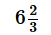days to complete the design.

QUESTION: 8

Working individually, Abe, Bosky, and Chris could do the same job in 12, 24 and 36 hours respectively. They decided to work in cycles of 3 hours with each person working exactly for an hour in a cycle. If they could start the work in any order and only one person works on the job at any given time, what was the minimum amount of time in which the job could be completed?

Solution:

Step 1: Question statement and Inferences

We are given the time in which three workers Abe, Bosky, and Chris can finish a piece of work. Abe takes 12 hours to complete the work, Bosky takes 24 hours whereas Chris completes the work in 36 hours.

Now, we can easily find their respective rates of work.

Let’s say the total amount of work is W. Thus, the rate of work of the three workers: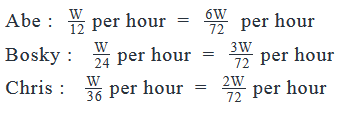Per the question statement the three workers work alternatively for an hour in turns. We have to find the minimum time in which the work can be completed:

Hence, only one of the workers will work on the job for an hour, then the second worker will work in place of him and then the third worker will replace him. This process continues till the job is completed.

Since any worker can start the job, the fastest worker should start the job to complete it in minimum time.

We know that Abe is the fastest worker, he finishes 1/12 part of the work in an hour, while Chris is the slowest, he finishes only 1/36 part of the work in an hour.

Thus, Abe should start the work, followed by Bosky followed by Chris.

Step 2: Finding required values

When each of Abe, Bosky, and Chris work for an hour, the amount of work completed at the end of the three hours: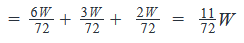After 6 rounds of working together, the total time consumed will be = 3*6 = 18 hours

And the total work completed will be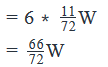Now, the remaining work is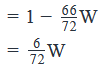Since now is Abe’s turn to work, he will complete the remaining 6/72W

in one hour.

Step 3: Calculating the final answer

So, the total number of hours taken to complete the job = 18 + 1 = 19 hours

QUESTION: 9

In how many days can 12 men and 15 women complete a job, if 8 men can do the same job in 12 days? Assume the rate of working of all men is same and rate of working of all women is same.

(1) 20 women can do the same job in 10 days.

(2) 24 men and 5 women can complete the same job in 40/11 days.

Solution:

The question is asking how long it would take 12 men and 15 women to complete a certain job. It tells you that 8 men complete this job in 12 days.

Let the total amount of work involved in completing the job be W.

Work done by 8 men in 12 days = W

Work done by 8 men in 1 day = W/12

Work done by 1 man in 1 day = W/96                      . . . Equation 1

So, work done by 12 men in 1 day = 12W/96 = W/8

Let one woman working alone complete the job in d days.

So, work done by 1 woman in 1 day =W/d                   . . . Equation 2

So, work done by 15 women in 1 day = 15W/d

Work done by 12 men and 15 women working together in 1 day = W/8 + 15W/d=(1/8+15/d)W

Once we know the value of d, we will be able to know the value of the coefficient of w in the above equation.

The reciprocal of this coefficient will be equal to the total time taken by 12 men and 15 women to complete the task.

So, we need to find the value of d.

Step 3: Analyze Statement 1

20 women can do the same job in 10 days.

Work done by 20 women in 10 days = W

Work done by 20 women in 1 day = W/10

Work done by 1 woman in 1 day =W/200                  . . . Equation 3

Comparing Equation 3 with Equation 2:

d=200

Thus, Statement 1 is sufficient to arrive at a unique value of the time taken by 12 men and 15 women

to complete the job

Step 4: Analyze Statement 2

24 men and 5 women can complete the same job in 40/11 days.

Work done by 24 men and 5 women in 40/11 days = W

Work done by 24 men and 5 women in 1 day =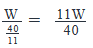Using Equations 1 and 2 we can write: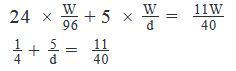Thus, we have a linear equation in d.

By solving this equation, we will be able to find the value of d

Thus, Statement 2 too is sufficient to arrive at a unique answer.

Step 5: Analyze Both Statements Together (if needed)

You get unique answers in steps 3 and 4, so this step is not required

QUESTION: 10

Suzy and Marie decide to help Leslie with her thesis. Suzy can type 3 chapters per hour, Leslie can type 5 chapters per hour, and Marie can type 6 chapters per hour. If the thesis contains 28 chapters, how many hours with it take the three of them, working together, to complete the thesis?

Solution:

Step 1: Question statement and Inferences

The question asks for the amount of time that the three friends, working together at their respective rates, will take to complete the thesis. You are given the rates of each friend, and you can infer that the rates are consistent. In other words, the women typing will consistently complete the same number of chapters per hour.

Step 2: Finding required values

Use the rates that the three friends type. Per each hour, Suzy, Marie, and Leslie type 3, 5, and 6 chapters each, respectively.  The thesis contains 28 chapters total.

Step 3: Calculating the final answer

In the first hour working together, Suzy, Marie, and Leslie type 3, 5, and 6 chapters each, thus typing a total of 14 chapters together. In the second hour, they type the same number of chapters, for a total of 14. At the end of this second hour, they have typed 28 chapters, which is the entire thesis.

Working together, it will take the three friends 2 hours to type the thesis.

QUESTION: 11

20 men can do a job in 5 days while 10 women can do the same job in 4 days. In how many days can 2 men and 8 women complete the job? Assume the rate of work of all men to be equal and the rate of work of all women to be equal.

Solution:

Step 1: Question statement and Inferences

This is a problem involving two different rates of work. We will first solve for these individual rates of work, and then, we will find the combined rate of work by adding the individual rates of work. Once we know the combined rate of work, we will be able to find the total time taken to complete the work.

Step 2: Finding required values

Let the total amount of work be W

Work done by 20 men in 5 days = W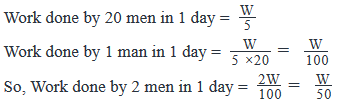Now considering the women:

Work done by 10 women in 4 days = W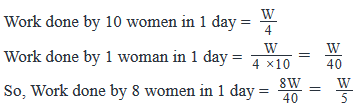So, work done by 2 men and 8 women in 1 day =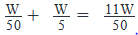Step 3: Calculating the final answer

Total time taken to complete W amount of work = 50/11  days

Looking at the answer choices, we see that Option D is correct

QUESTION: 12

Jeff was given a report to complete. In how many days can he complete the report, if his rate of completion is consistent?

(1) Jeff can complete 10% of the report in 2 days.

(2) After 7 days, there was still 65% of the report to be completed.

Solution:

Steps 1 & 2: Understand Question and Draw Inferences

The question asks for the number of days it will take Jeff to complete the report. You need to know the portion of the report, whether as a fraction or a percent, that Jeff can complete in a single day.

We are also given that he completes the same percent of his report every day.

Step 3: Analyze Statement 1

(1) Jeff can complete 10% of the report in 2 days.

If Jeff completes 10% of the report in 2 days, then he completes 5% in one day. At this rate, it will take Jeff 20 days to complete the report.

Statement 1 is sufficient.

Step 4: Analyze Statement 2

(2) After 7 days, there was still 65% of the report to be completed.

If 65% of the report remains after 7 days, then Jeff has completed 35% during these 7 days. AT this rate, he completes 5% in one day, and it will take him 20 days to complete the report.

Statement 2 is sufficient.

Step 5: Analyze Both Statements Together (if needed)

You get unique answers in steps 3 and 4, so this step is not required

QUESTION: 13

P is able to do a piece of work in 15 days and Q can do the same work in 20 days. If they can work together for 4 days, what is the fraction of work left?

Solution:

Amount of work P can do in 1 day = 1/15
Amount of work Q can do in 1 day = 1/20
Amount of work P and Q can do in 1 day = 1/15 + 1/20 = 7/60
Amount of work P and Q can together do in 4 days = 4 × (7/60) = 7/15
Fraction of work left = 1 – 7/15= 8/15

QUESTION: 14

P can lay railway track between two stations in 16 days. Q can do the same job in 12 days. With the help of R, they completes the job in 4 days. How much days does it take for R alone to complete the work?

Solution:

Amount of work P can do in 1 day = 1/16
Amount of work Q can do in 1 day = 1/12
Amount of work P, Q and R can together do in 1 day = 1/4
Amount of work R can do in 1 day = 1/4 - (1/16 + 1/12) = 3/16 – 1/12 = 5/48
=> Hence R can do the job on 48/5 days = 9 (3/5) days

QUESTION: 15

P, Q and R can do a work in 20, 30 and 60 days respectively. How many days does it need to complete the work if P does the work and he is assisted by Q and R on every third day?​

Solution:

Amount of work P can do in 1 day = 1/20
Amount of work Q can do in 1 day = 1/30
Amount of work R can do in 1 day = 1/60
P is working alone and every third day Q and R is helping him
Work completed in every three days = 2 × (1/20) + (1/20 + 1/30 + 1/60) = 1/5
So work completed in 15 days = 5 × 1/5 = 1
i.e, the work will be done in 15 days